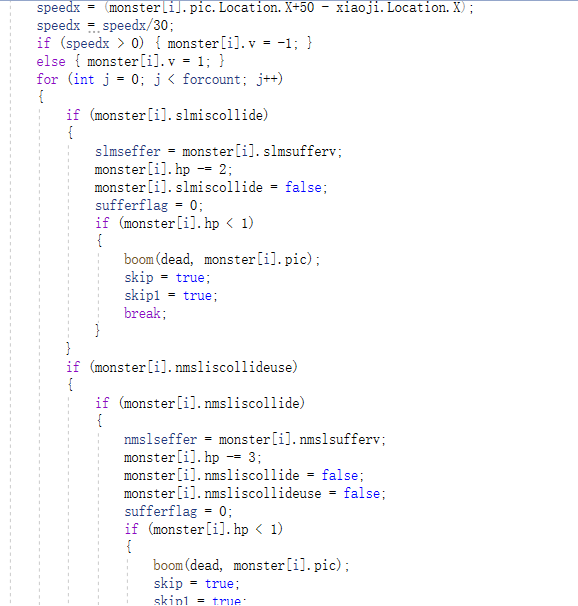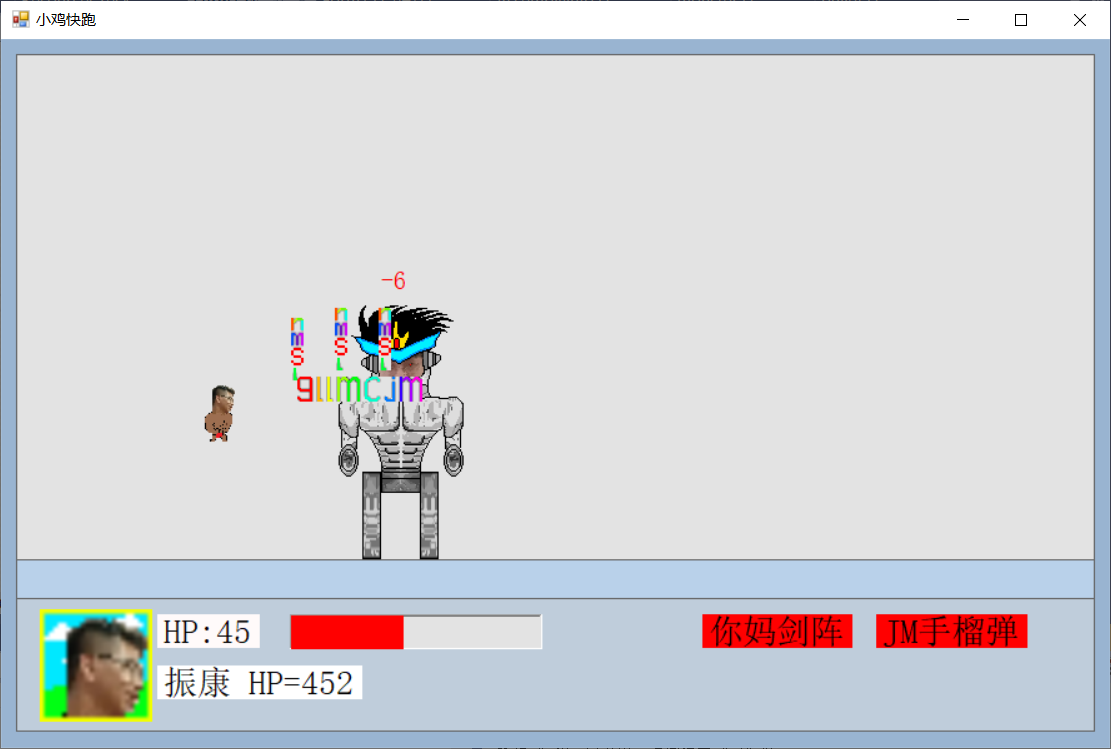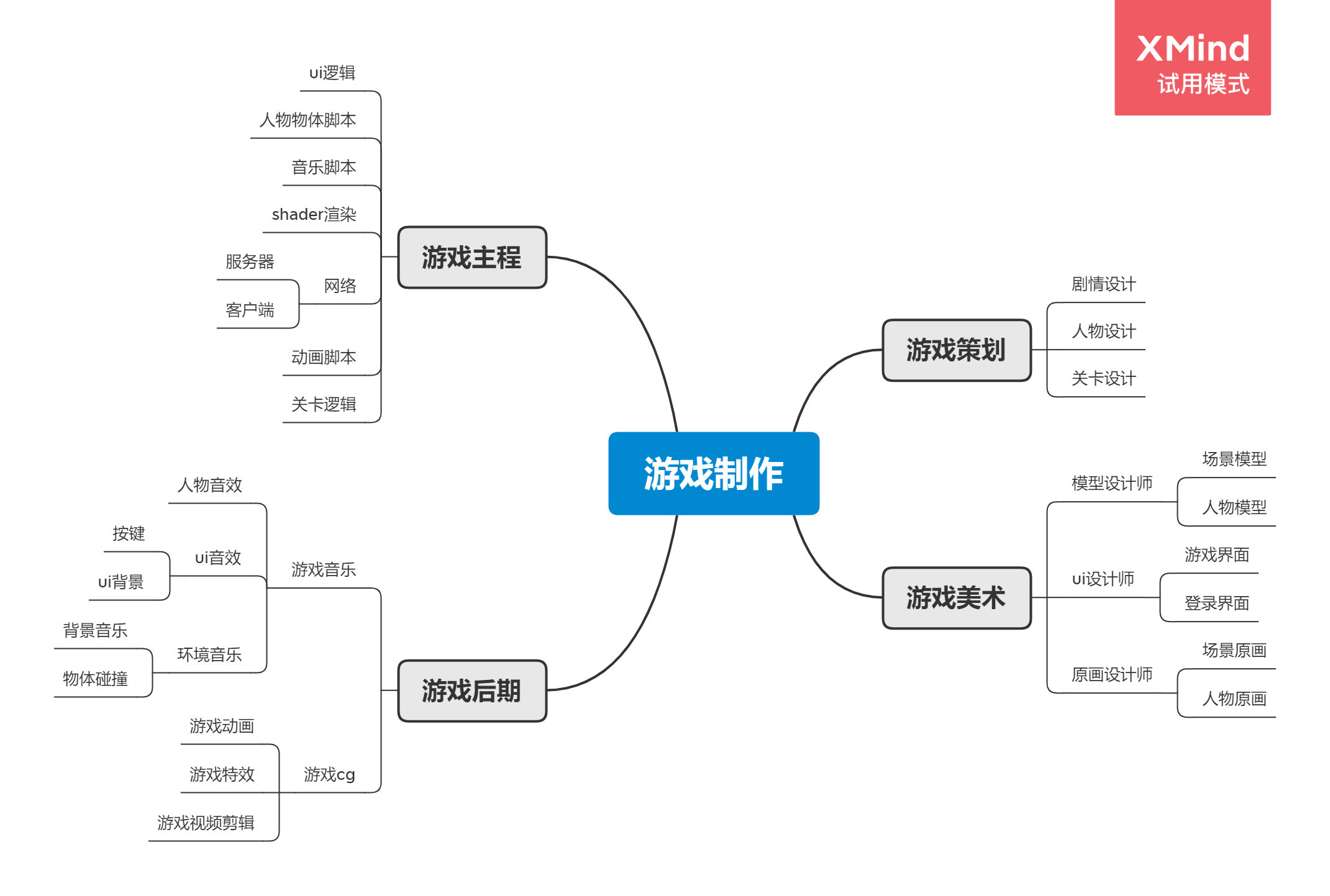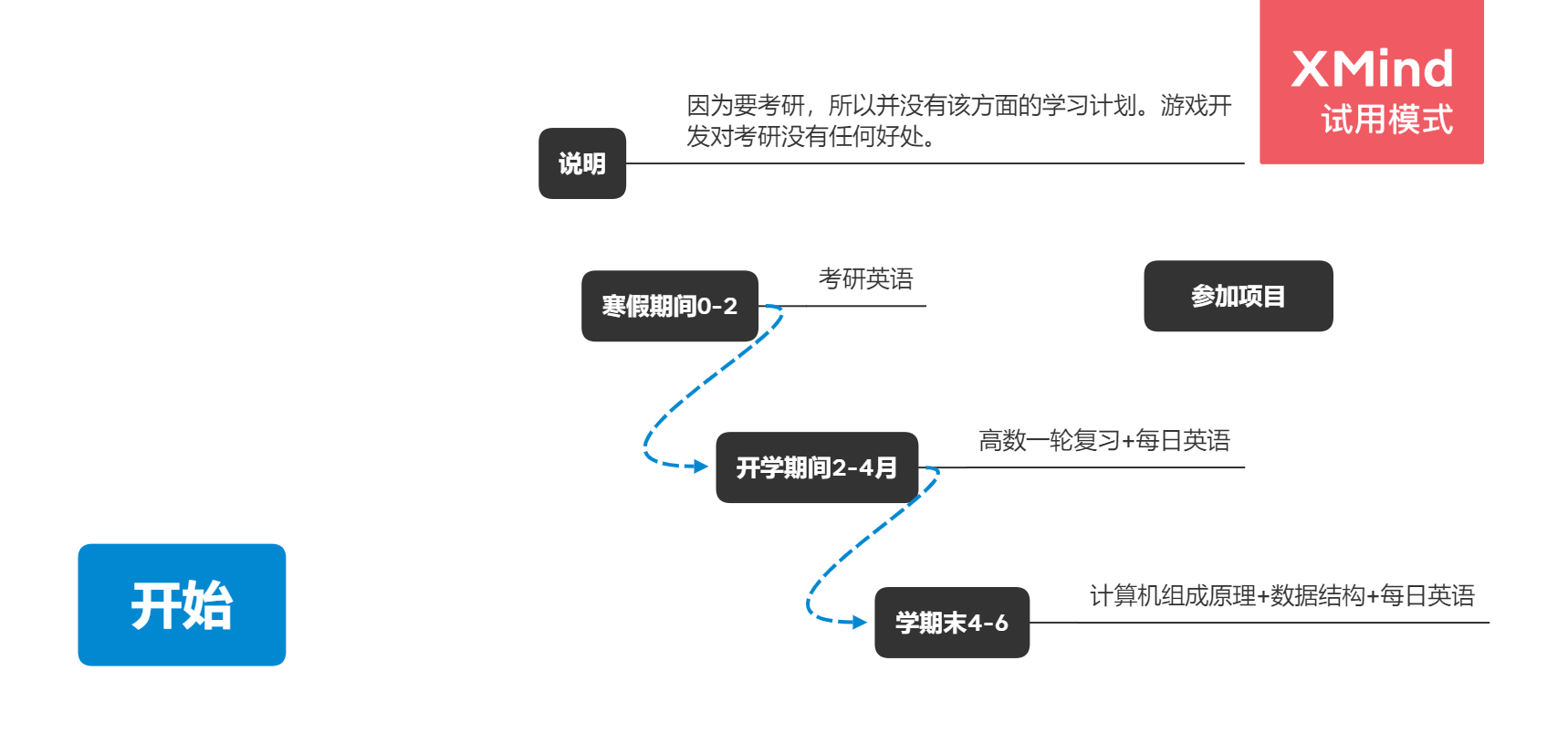# 寒假作业1/2

## 2. 立足当下

### 1.个人信息姓名 潘某

### 2.当前值

#### 3.自主开发的小游戏

for (int i = 0; i < 13; i++) { if (monster[i].isalive) { for (int j = 0; j < 10; j++) { if (slmisfly[j]) { if (iscollide(monster[i].pic, slm[j])) { slmiscollide[j] = true; monster[i].slmiscollide = true; monster[i].slmsufferv = slmv[j]; } } } for(int j=0;j<4;j++) { if (!nmsluseflag) { if (iscollide(monster[i].pic, nmsl[j])) { monster[i].nmsliscollide = true; monster[i].nmslsufferv = nmslv; } } } if (iscollide(monster[i].pic, gllmcjm)|| iscollide(monster[i].pic, boom1)|| iscollide(monster[i].pic, boom2)) { if (!bigflag) { monster[i].gllmcjmiscollide = true; monster[i].gllmcjmsufferv = gllmcjmv; } } }## 3. 展望未来

### 1. 阅读《构建之法》，并提出3个及以上的问题。

1.作为一个名软件工程师，他的成长历程是什么？
2.软件的定义是什么？
3.软件的质量是如何去衡量的？
4.软件代码是开源还是不开源好。

## 4. 思维导图和学习路线

### 1.思维导图### 2.学习计划posted @ 2021-02-02 17:35  要记得吃饭啊  阅读(56)  评论(4编辑  收藏